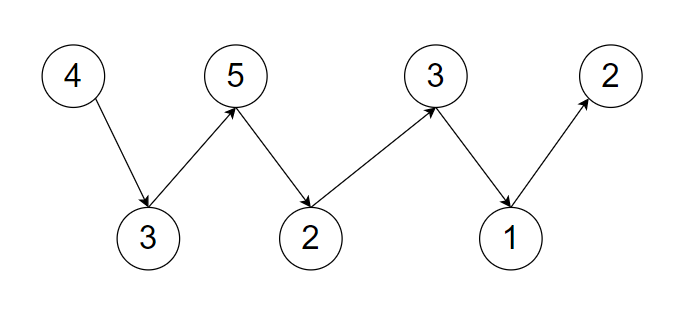New update is available. Click here to update.

# Sort an array in wave form

Posted: 9 Dec, 2020
Difficulty: Easy

## PROBLEM STATEMENT

#### Your task is to sort the array in such a way that the array looks like a wave array.

##### Example:
``````If the given sequence ‘ARR’ has ‘N’ elements then the sorted wave array looks like -
‘ARR >= ARR’ and ‘ARR <= ARR’
‘ARR >= ARR’ and ‘ARR <= ARR’
‘ARR >= ARR’ and ‘ARR <= ARR’  And so on.
``````
##### Note:
``````1. ‘ARR’ must be greater than or equal to ‘ARR’.

2. There can be multiple arrays that look like a wave array but you have to return only one.

3. We have an internal function that will check your solution and return 'True' in case your array is one of the solutions otherwise return 'False'.
``````

#### Explanation

``````The given array ‘ ARR = { 4, 3, 5, 2, 3, 1, 2 } ’
The below figure is a visual representation of the given ‘ARR’ and you can see we can express ‘ARR’ in a waveform array because
4>3 and 3<5
5>2 and 2<3
3>1 and 1<2
And it follows the condition of wave array.
````````````Try to solve this problem in linear time complexity.
``````
##### Input format:
``````The first line of input contains an integer ‘T’ denoting the number of test cases.
The next ‘2*T’ lines represent the ‘T’ test cases.

The first line of each test case contains the single integer ‘N’ and ‘N’ is denoting the number elements in the given ‘ARR’.

The second line of each test case contains ‘N’ space-separated elements of ‘ARR’.
``````
##### Output Format:
``````For each test case, print in a single line space-separated integers that represent the elements of a wave array.
``````
##### Note:
``````You are not required to print the output explicitly, it has already been taken care of. Just implement the function.
``````
##### Constraints:
``````1 <= T <= 50
1 <= N<= 10^4
-10^9 <= ARR[i] <= 10^9

Time limit: 1 second
``````
SIMILAR PROBLEMS

Find minimum

Posted: 8 Nov, 2022
Difficulty: Hard

Merge Two Sorted Arrays Without Extra Space

Posted: 19 Nov, 2022
Difficulty: Moderate

Search In A Sorted 2D Matrix

Posted: 23 Nov, 2022
Difficulty: Moderate

Sort 0s, 1s, 2s

Posted: 24 Dec, 2022
Difficulty: Easy

Fake Coin Problem

Posted: 24 Dec, 2022
Difficulty: Easy